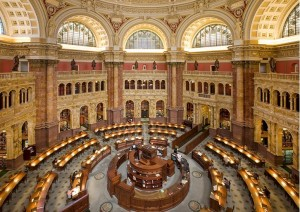A Universal LibraryMain Reading Room, Library of Congress: Image Carol M. Highsmith There is much literature on the topic of an infinite library. It will contain all books that have ever been written and all books that will be written. So if you are going to create such a universal library suppose you allow for each book

• 26 letters,
• 10 numerical digits,
• 10 characters for punctuation,
• A space,
• A line length of 100,
• The number of lines per page of 50,
• The number of pages per book of 200.
The total number of books in a universal library would be

(26 + 10 + 10 + 1) x 100 x 50 x 200 = 470,000,000

If you allowed a one cubic inch of space for each book you would need 470,000,000 cubic inches, or 271990.7407 cubic feet.

The books would fit into a room about 100 feet long x 100 wide x 28 feet high.

If you say that 200 pages are not enough for some books, I allow multiple volumes.

Of course, most of the books would be nonsense. There is one book of only blanks, one book with “0” on the first line of the first page with blanks filling the rest of the book. There would be many books differing by just one space, many just by two, etc. There would be many books about your birth. In many books you would have the same parents; in other books they would be different (How about Einstein and Madonna?). You would be born in many different places on many different dates. One can now begin to see that the library is useless. You would have to sort through many books to find a book that was not gibberish.

That being said, let us move the situation to the reductio ad absurdum level. In writing out text for the books in ASCII, American Standard Code for Information Exchange, we can reduce the number of books drastically. Each of the letters, numerical digits, characters for punctuation and the space can be written using only zeros and ones. For instance

“A” can be written as 01000001

And this being said, we can reduce the number of books to two:

• 2 symbols, 0 and 1
• A line length on 1
• The number of lines per page is 1
• And the number of pages per book is 1
The first book contains a zero; the second book contains a 1. Any book in the old sense can be realized by the appropriate number of volumes.

So there are 8 volumes containing the letter “A”:

The first book contains a 0, the second book contains a 1, the third book contains a 0 … You get the idea. An interesting fictional treatment of the idea of an infinite library can be found in the short story, The Library of Babel, by Jorge Luis Borges.

-Ellen Hetland Fenwick: A Universal Library

References: ← Back to Math-Logic-Design
← Home